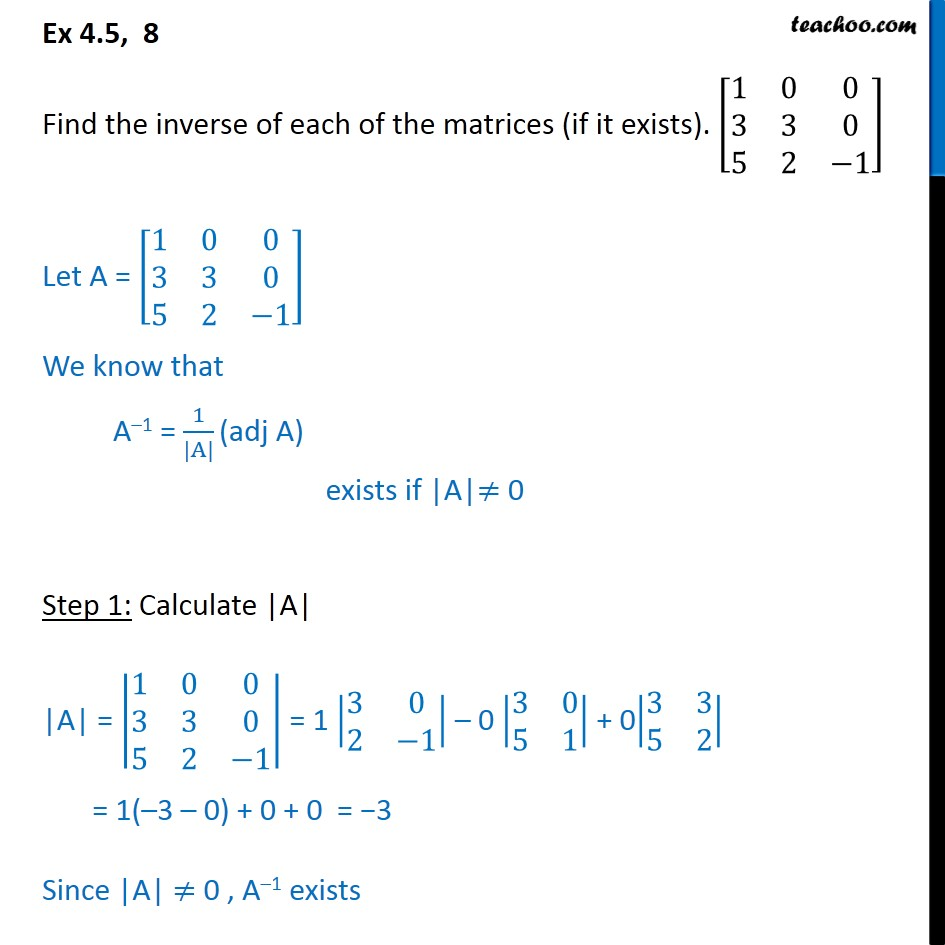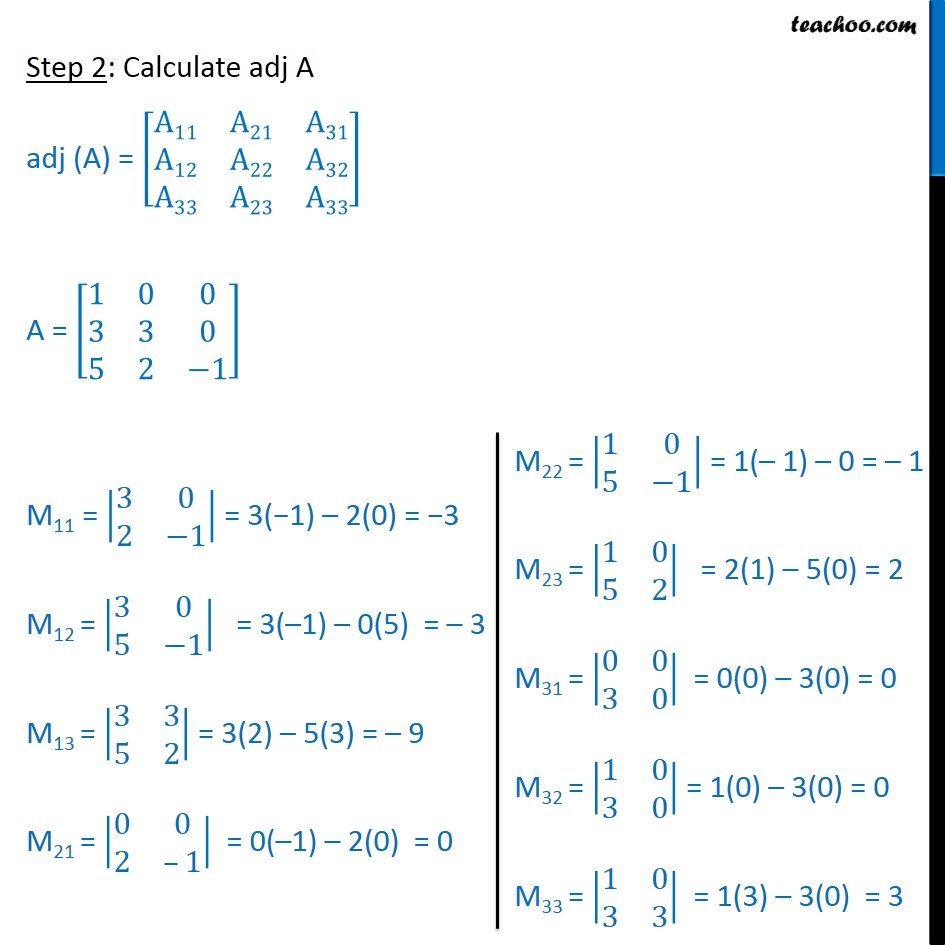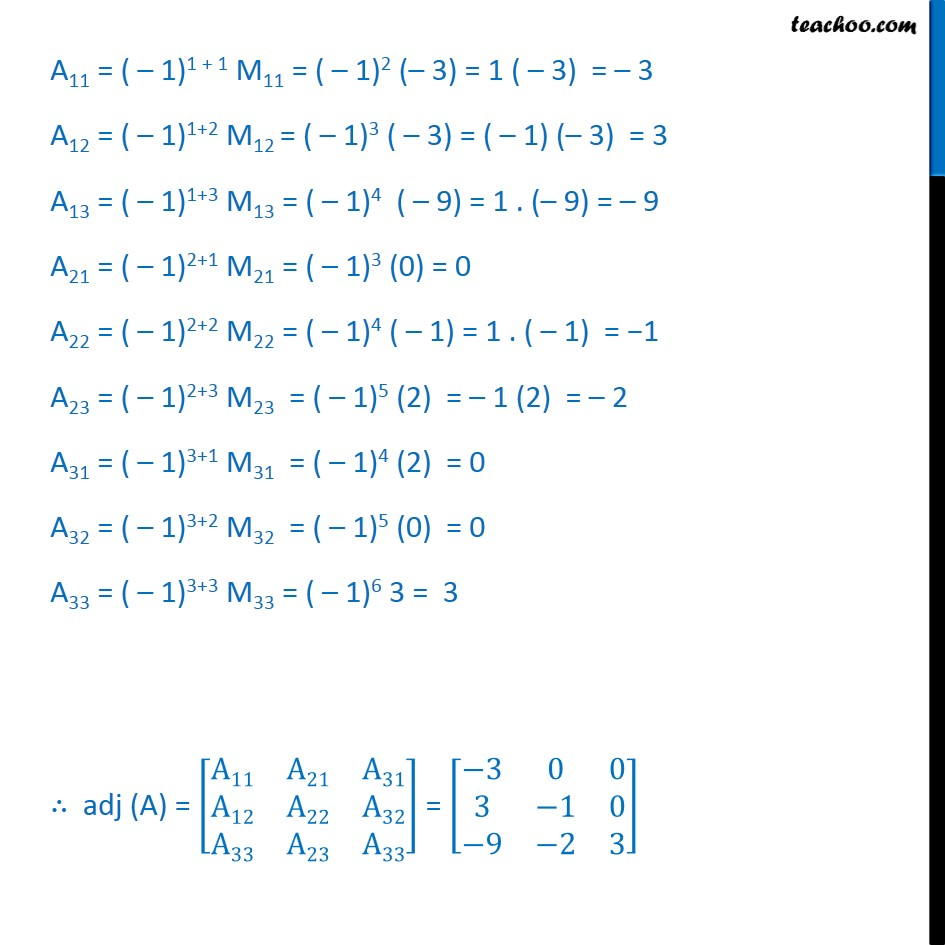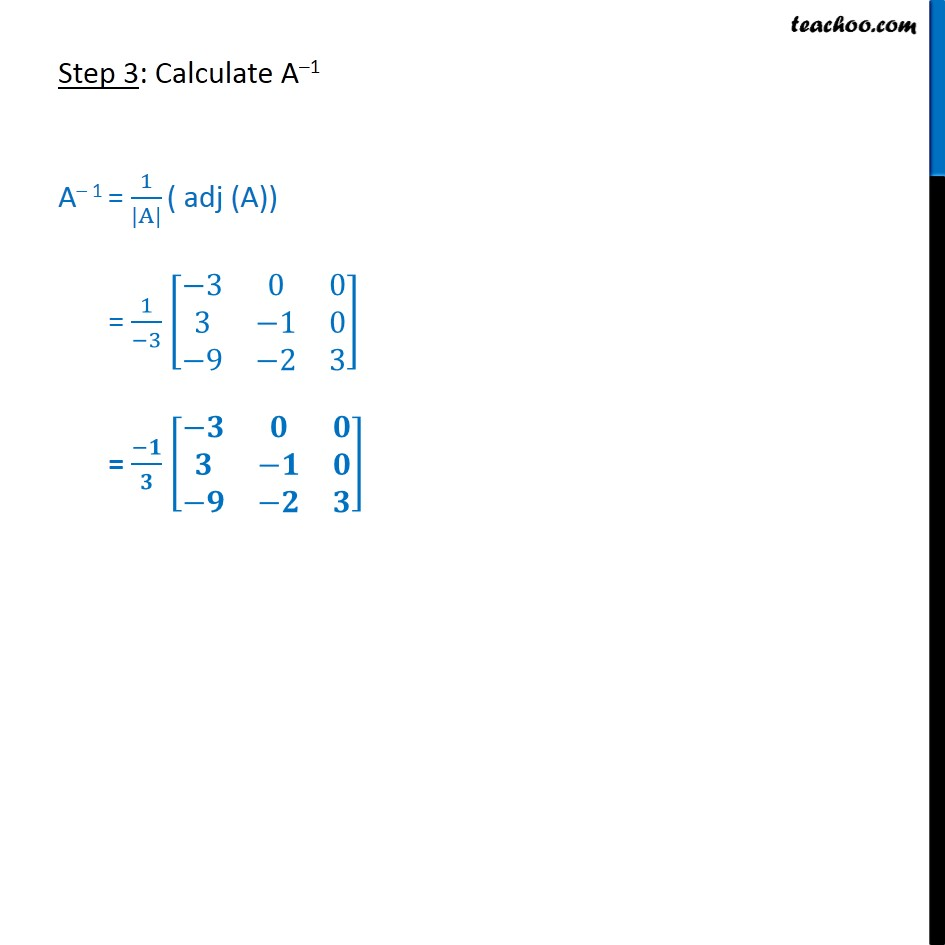Ex 4.5

Chapter 4 Class 12 Determinants
Serial order wiseGet live Maths 1-on-1 Classs - Class 6 to 12

### Transcript

Ex 4.5, 8 Find the inverse of each of the matrices (if it exists). 1﷮0﷮0﷮3﷮3﷮0﷮5﷮2﷮−1﷯﷯ Let A = 1﷮0﷮0﷮3﷮3﷮0﷮5﷮2﷮−1﷯﷯ We know that A–1 = 1﷮|A|﷯ (adj A) exists if |A|≠ 0 Step 1: Calculate |A| |A| = 1﷮0﷮0﷮3﷮3﷮0﷮5﷮2﷮−1﷯﷯ = 1 3﷮0﷮2﷮−1﷯﷯ – 0 3﷮0﷮5﷮1﷯﷯ + 0 3﷮3﷮5﷮2﷯﷯ = 1(–3 – 0) + 0 + 0 = −3 Since |A| ≠ 0 , A–1 exists Step 2: Calculate adj A adj (A) = A11﷮A21﷮A31﷮A12﷮A22﷮A32﷮A33﷮A23﷮A33﷯﷯ A = 1﷮0﷮0﷮3﷮3﷮0﷮5﷮2﷮−1﷯﷯ M11 = 3﷮0﷮2﷮−1﷯﷯ = 3(−1) – 2(0) = −3 M12 = 3﷮0﷮5﷮−1﷯﷯ = 3(–1) – 0(5) = – 3 M13 = 3﷮3﷮5﷮2﷯﷯ = 3(2) – 5(3) = – 9 M21 = 0﷮0﷮2﷮–1﷯﷯ = 0(–1) – 2(0) = 0 A11 = ( – 1)1 + 1 M11 = ( – 1)2 (– 3) = 1 ( – 3) = – 3 A12 = ( – 1)1+2 M12 = ( – 1)3 ( – 3) = ( – 1) (– 3) = 3 A13 = ( – 1)1+3 M13 = ( – 1)4 ( – 9) = 1 . (– 9) = – 9 A21 = ( – 1)2+1 M21 = ( – 1)3 (0) = 0 A22 = ( – 1)2+2 M22 = ( – 1)4 ( – 1) = 1 . ( – 1) = −1 A23 = ( – 1)2+3 M23 = ( – 1)5 (2) = – 1 (2) = – 2 A31 = ( – 1)3+1 M31 = ( – 1)4 (2) = 0 A32 = ( – 1)3+2 M32 = ( – 1)5 (0) = 0 A33 = ( – 1)3+3 M33 = ( – 1)6 3 = 3 ∴ adj (A) = A11﷮A21﷮A31﷮A12﷮A22﷮A32﷮A33﷮A23﷮A33﷯﷯ = −3﷮0﷮0﷮3﷮−1﷮0﷮−9﷮−2﷮3﷯﷯ Step 3: Calculate A–1 A– 1 = 1﷮|A|﷯ ( adj (A)) = 1﷮−3﷯ −3﷮0﷮0﷮3﷮−1﷮0﷮−9﷮−2﷮3﷯﷯ = −𝟏﷮𝟑﷯ −𝟑﷮𝟎﷮𝟎﷮𝟑﷮−𝟏﷮𝟎﷮−𝟗﷮−𝟐﷮𝟑﷯﷯